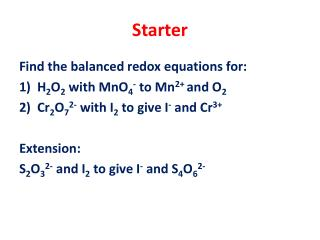DownloadDownload PresentationStarter

# Starter

Download Presentation## Starter

- - - - - - - - - - - - - - - - - - - - - - - - - - - E N D - - - - - - - - - - - - - - - - - - - - - - - - - - -
##### Presentation Transcript

1. Starter Find the balanced redox equations for: • H2O2 with MnO4- to Mn2+ and O2 • Cr2O72- with I2 to give I- and Cr3+ Extension: S2O32- and I2 to give I- and S4O62-

2. Starter Find the balanced redox equations for: • H2O2 with MnO4- to Mn2+ and O2 MnO4¯ + 5e¯ + 8H+ —> Mn2+ + 4H2O(x2) H2O2——> O2 + 2H+ + 2e¯ (x5) 2MnO4¯ + 5H2O2 + 6H+ —> 2Mn2+ + 5O2 + 8H2O

3. Starter Find the balanced redox equations for: Cr2O72- with I2 to give I- and Cr3+ Cr2O72- + 14H+ + 6e¯ —> 2Cr3+ + 7H2O (x1) ½ I2 + e¯ —> I¯(x6) Cr2O72- + 14H+ + 3I2 —> 2Cr3+ + 6I ¯ + 7H2O

4. Starter S2O32-and I2 to give I- and S4O62- • 2S2O32- ——> S4O62- + 2e¯ (x1) • ½ I2 + e¯ ——> I¯(x2) • 2S2O32- + I2 ——> S4O62- + 2I¯

5. Cell Potential L.O.: Define the term standard electrode potential. Describe how to make an electrochemical cell. Calculate the standard cell potential of a cell.

6. Homework

7. Electrode Potentials • know the IUPAC convention for writing half-equations for electrode reactions. • Know and be able to use the conventional representation of cells. • Know that standard electrode potential, E , refers to conditions of 298 K, 100 kPa and 1.00 mol dm−3 solution of ions.

8. Half-cell: an element in two oxidation states.

9. Zn2+(aq) + 2 e–  Zn(s)

10. Zn2+(aq) + 2e¯ ->Zn(s) E° = - 0.76V The electrode potential of the half cell indicates its tendency to lose or gain electrons.

11. The standard electrode potential of a half-cell, is the e.m.f of a cell compared with a standard hydrogen half-cell, measured at 298 K with a solution concentration of 1 mol dm-3 and a gas pressure of 100KPa

12. Standard Conditions Concentration 1.0 mol dm-3 (ions involved in ½ equation) Temperature 298 K Pressure 100 kPa(if gases involved in ½ equation) Current Zero (use high resistance voltmeter)

13. S tandard H ydrogen E lectrode

14. - electrode anode oxidation + electrode cathode reduction electron flow At this electrode the metal loses electrons and so is oxidised to metal ions. These electrons make the electrode negative. At this electrode the metal ions gain electrons and so is reduced to metal atoms. As electrons are used up, this makes the electrode positive. Zn Cu Zn  Zn2+ + 2 e- oxidation Cu2+ + 2 e- Cu reduction

15. Emf = E = E(positive terminal) - E(negativeterminal)

16. Pt(s) | H2(g) | H+(aq) || Cu2+(aq) | Cu(s)

17. GOLDEN RULE The more +ve electrode gains electrons (+ charge attracts electrons)

18. Electrodes with negative emf are better at releasing electrons (better reducing agents).

19. A2.CHEM5.3.003 5.3 EXERCISE 2 - electrochemical cells

20. ELECTRODE POTENTIALS – Q1 Emf = Eright - Eleft - 2.71 = Eright - 0 Eright = - 2.71 V

21. ELECTRODE POTENTIALS – Q2 Emf = Eright - Eleft Emf = - 0.44 - 0.22 Emf= - 0.66 V

22. ELECTRODE POTENTIALS – Q3 Emf = Eright - Eleft Emf = - 0.13 - (-0.76) Emf= + 0.63 V

23. ELECTRODE POTENTIALS – Q4 Emf = Eright - Eleft +1.02 = +1.36 - Eleft Eleft = + 1.36 - 1.02 = +0.34 V

24. ELECTRODE POTENTIALS – Q5 Emf = Eright - Eleft a) Emf = + 0.15 - (-0.25) = +0.40 V b) Emf = + 0.80 - 0.54 = +0.26 V c) Emf = + 1.07 - 1.36 = - 0.29 V

25. ELECTRODE POTENTIALS – Q6 Emf = Eright - Eleft a) Eright = +2.00 - 2.38 = - 0.38 V Ti3+(aq) + e- Ti2+(aq) b) Eleft = -2.38 - 0.54 = - 2.92 V K+(aq) + e- K(aq) c) Eright = - 3.19 + 0.27 = - 2.92 V Ti3+(aq) + e- Ti2+(aq)

26. ELECTRODE POTENTIALS – Q7 a) Cr(s) | Cr2+(aq) || Zn2+(aq) | Zn(s) Emf = -0.76 - (-0.91) = +0.15 V b) Cu(s) |Cu2+(aq)|| Fe3+(aq),Fe2+(aq)| Pt(s) Emf = +0.77 - 0.34 = +0.43 V c) Pt(s) | Cl-(aq)| Cl2(g) || MnO4-(aq),H+(aq),Mn2+(aq)| Pt(s) Emf = +1.51 – 1.36 = +0.15 V